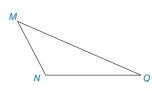Chapter 2.1, Problem 34E### Elementary Geometry for College St...

6th Edition
Daniel C. Alexander + 1 other
ISBN: 9781285195698

#### Solutions

Chapter
Section### Elementary Geometry for College St...

6th Edition
Daniel C. Alexander + 1 other
ISBN: 9781285195698
Textbook Problem
92 views

# Given: Triangle M N Q with obtuse ∠ M N Q Construct: N E ¯ ⊥ M Q ¯ , with E on M Q ¯ .Exercise 36, 37

To determine

To construct:

The perpendicular line on the given line.

Explanation

Given:

Triangle MNQ with obtuse MNQ.

The give line is MQ¯.

The given figure is,

Approach:

The given figure is,

Figure (1)

Use compass to make an arc from N that intersect MQ at point A and B as shown in figure (2).

Figure (2)

With A and B as center mark two arcs equal radii NB to intersect at point L as shown in figure (3)

### Still sussing out bartleby?

Check out a sample textbook solution.

See a sample solution

#### The Solution to Your Study Problems

Bartleby provides explanations to thousands of textbook problems written by our experts, many with advanced degrees!

Get Started

#### Find more solutions based on key concepts